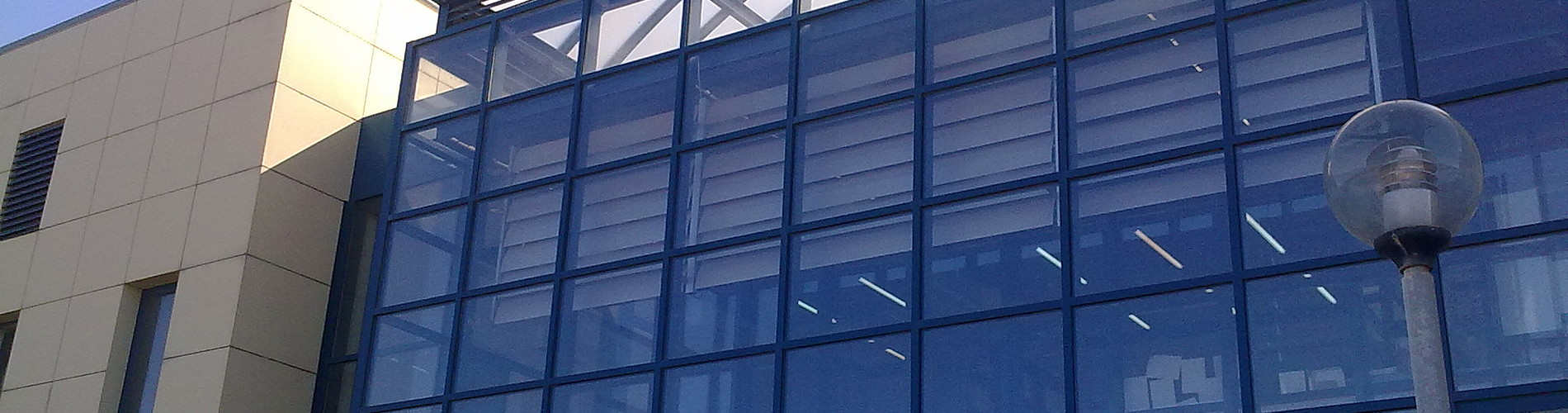School of Chemical and Environmental Engineering

Now offering two distinct diplomas: Chemical Engineering and Environmental Engineering

# Linear Algebra

1. COURSE INFORMATION:

 School Chemical and Environmental Engineering Course Level Undergraduate Direction - Course ID MATH 201 Semester 3rd Course Category Required Course Modules Instruction Hours per Week ECTS Lectures and Tutorial Exercises 5 Th=4, E=1, L=0 4 Course Type General Background Prerequisites Instruction/Exam Language Greek The course is offered to Erasmus students No Course URL https//www.eclass.tuc.gr/courses/MHPER313/  (in Greek)

2. LEARNING OUTCOMES

 Learning Outcomes The goal of the course is to introduce the basic fundamental ideas and techniques of Linear Algebra, ideas the students will meet in many other basic and advanced courses .The course begins with the algebra of matrices and vectors and then considers methods of solution of Linear systems (some of them are produced by the modeling of physical phenomena).The notions of the inverse and the determinant of a matrix are presented. The notion of vector space (and subspace) is introduced and we learn how these notions are related to linear systems. The notions of linear independents, basis and dimension are defined. We discuss complemented subspaces, and how to find projections onto them. The notions of eigenvalue and eigenvector is introduced. We study the diagonalization of a matrix and the method of least squaresThe last section contains the basic recursive methods of solution of linear systems. With the successful completeness of the course the student is in a position  to:Recognize notions, properties and operations with vectors  and matrices. Determine in which class belong certain matrices(square, non-square, symmetric, orthogonal, positive definite, invertible  etc.)Understand the basic notions of linear independence of vectors, the concept of basis and the linear hull of a set of vectors.Solve singular or non-singular linear systems with the elimination methods/algorithms.Apply basic techniques of linear algebra in order to find the inverse of a matrix.Calculate the null space, the column space and the line space and the rank of a matrix  and to relate them with linear systems of equations.Calculate the determinant of a square matrix,  the characteristic polynomial, the eigenvalues and the eigenvectors of it, and in case this can be done to be able to find the diagonal form of a matrix.Apply the  Gram-Schmidt orthonormalization and orthonormalize the basis of a vector space of finite dimension.   .Recognize projections and complemented subspaces in spaces of finite dimension.Use ,  Cramer’s law, the nethod of least squares, simple Markov chains General Competencies/Skills Independent work

3. COURSE SYLLABUS

 Introduction to Linear Algebra.Algebra of matrices and vectors.Immediate methods of solution of linear systems.Determinants.Vector spaces, subspaces.Linear independence of vectors, basis of a space.Fundamental subspaces of a matrix.Eigenvalues and eigenvectors.Diagonalization and applications.Gram-Schmidt orthonormality.The method of least squares.Recursive methods of solution of linear systems.Exercises

4. INSTRUCTION and LEARNING METHODS - ASSESSMENT

 Lecture Method Direct (face to face) Use of Information and Communication Technology Specialized software, E-class support Instruction Organisation Activity Workload per Semester (hours) - Lectures 52 - Lab assignments 13 - Autonomous study 35 Course Total 100 Assessment Method Written final examination (100%) orWritten final examination (85%) and midterm exams (15%)